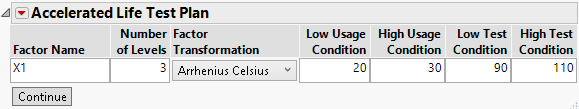Publication date: 06/16/2020

## Specify Acceleration Factors

Specify details about the acceleration factor or factors.

Figure 22.17 ALT Specification WindowFactor Name

Enter a name for each acceleration factor.

Number of Levels

For each acceleration factor, enter the number of proposed levels that you want to include in the experiment.

Factor Transformation

For each acceleration factor, select a transformation function. This transformation describes the life-stress relationship, which is the manner in which the life distribution changes across stress levels. The transformations available are Arrhenius Celsius, Reciprocal, Log, Square Root, and Linear.

Low Usage Condition

For each acceleration factor, enter a lower bound for its value in typical usage conditions.

High Usage Condition

For each acceleration factor, enter an upper bound for its value in typical usage conditions.

Note: The Low Usage Condition and High Usage Condition values can be equal. Use equal values when the usage condition is a single value.

Low Test Condition

For each acceleration factor, enter the low test condition.

High Test Condition

For each acceleration factor, enter the high test condition.

Note: The low and high test conditions define the endpoints of the acceleration factors in the design. The initial candidate will use equally spaced levels between the low and high test conditions. You can adjust the levels before constructing the design.